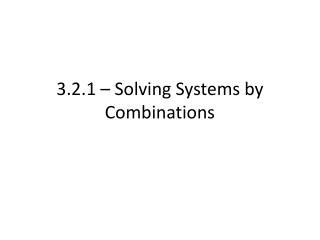# 3.2.1 – Solving Systems by Combinations - PowerPoint PPT PresentationDownload Presentation3.2.1 – Solving Systems by Combinations

3.2.1 – Solving Systems by Combinations
Download Presentation## 3.2.1 – Solving Systems by Combinations

- - - - - - - - - - - - - - - - - - - - - - - - - - - E N D - - - - - - - - - - - - - - - - - - - - - - - - - - -
##### Presentation Transcript

1. 3.2.1 – Solving Systems by Combinations

2. We have addressed the case of using substitution with linear systems • When would substitution not be easy to use?

3. Combinations • Similar to substitution, we can use a new method when solving for a specific variable may not be easy • Fractions • Multi-step • Odd Numbers

4. In order to use combinations, our goal is the following; • “Knock out” or eliminate one variable. Solve for the remaining. Then, similar to substitution, go back and find the other missing variable

5. How to use • To use the combination, or knock-out method, we do the following • 1) Find the variable with the same coefficient in both equations; multiply to get the same coefficient if necessary • 2) Add or subtract down, make sure one variable is eliminated • 3) solve for the remaining variable • 4) Go back to one of the original equations, and solve for the remaining variable • 5) Check final solutions

6. To help, it’s generally easiest to line the equations up as if you were doing addition or subtraction like you first learned • Add = if signs are opposite • Subtract = if signs are same

7. Example. Solve the following system. • 4x – 6y = 24 • 4x – 5y = 8

8. Example. Solve the following system. • 2x – 8y = 10 • -2x – y = -1

9. Example. Solve the following system. • 3x – y = -3 • x + y = 3

10. Multiplying • As mentioned, sometimes the coefficients may not be the same • Allowed to multiply one, or both equations, by a number to get the same coefficients for one of the variables • Make sure to multiple every term!

11. Example. Solve the following system. • 3x + 2y = -2 • x – y = 11 • Which variable should we try to cancel?

12. Example. Solve the following system. • 5x – 2y = -2 • 3x + 5y = 36

13. Assignment • Pg. 142 • 2, 4-6, 9-25 odd • Pg. 143 • 38, 39